# mathspresentation-160419194459.pdf

12 Sep 2022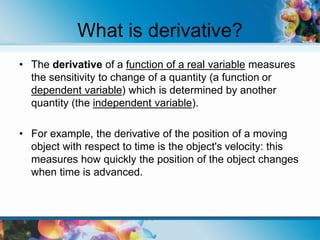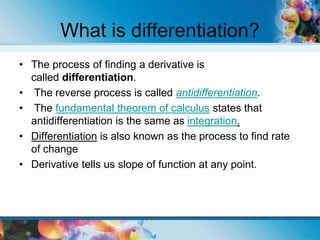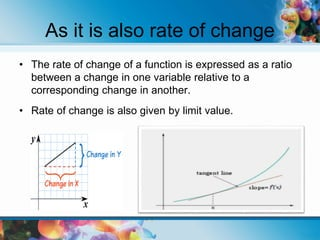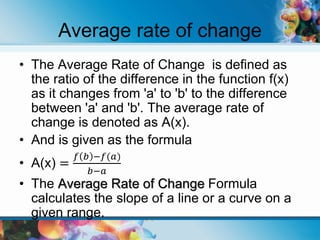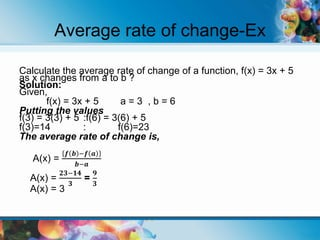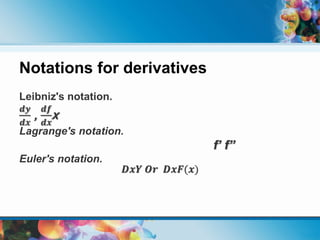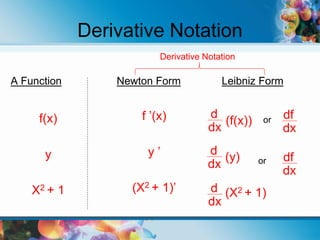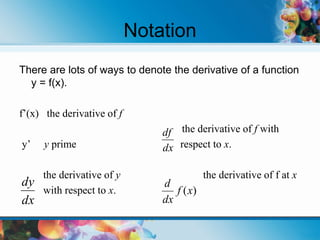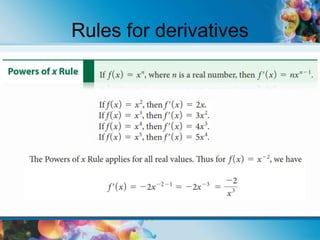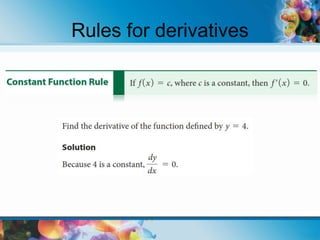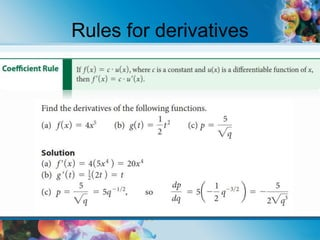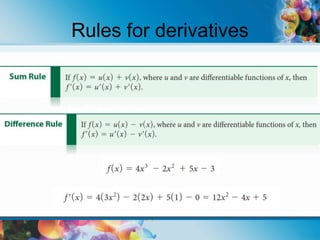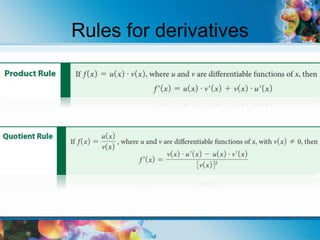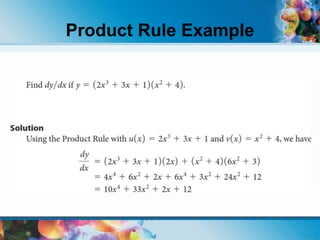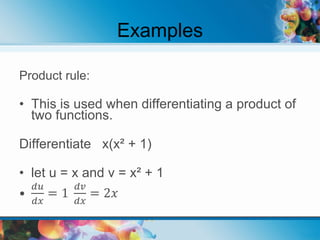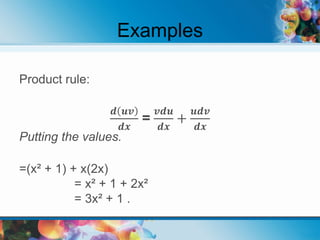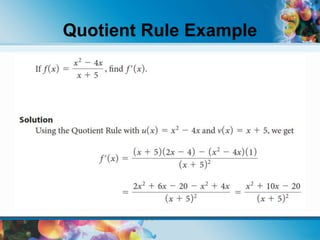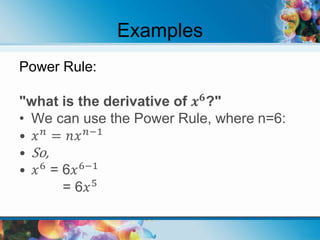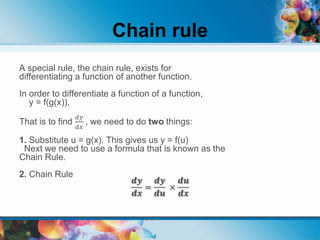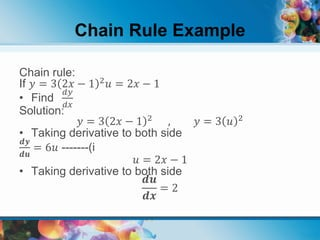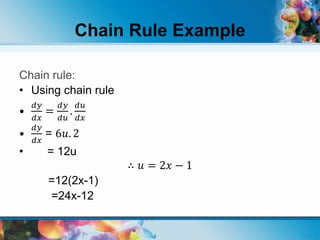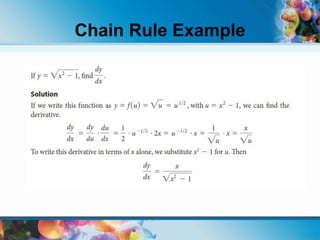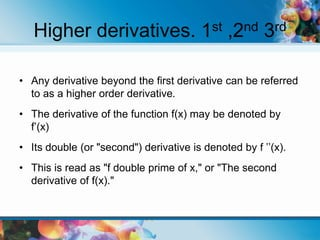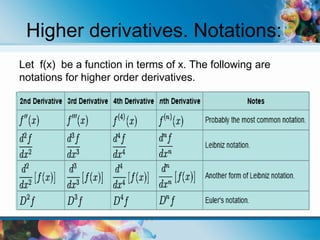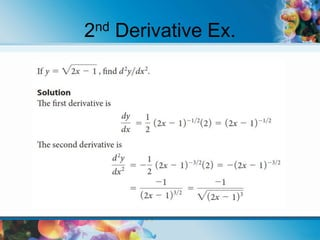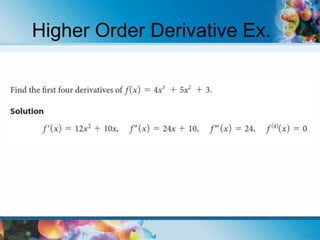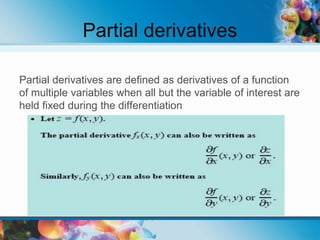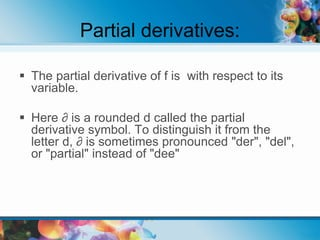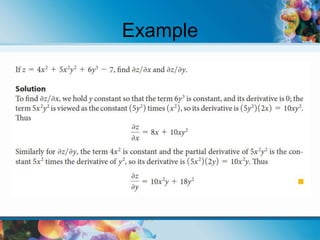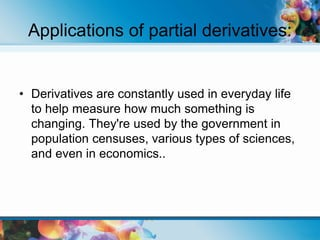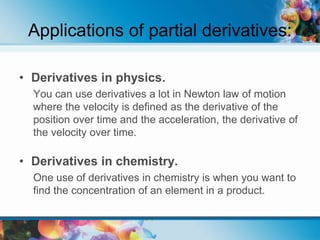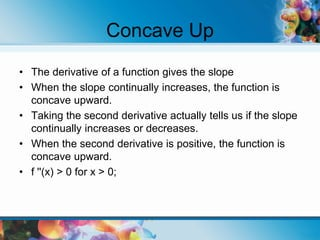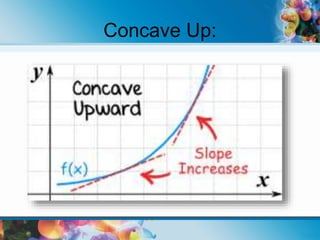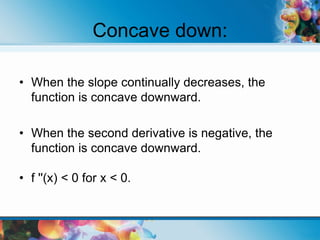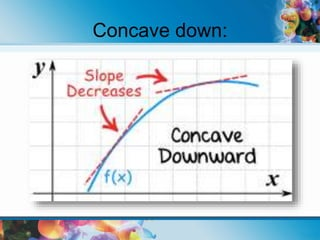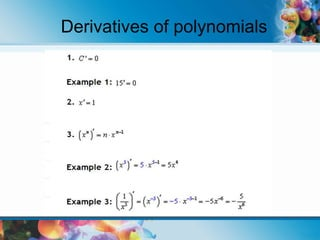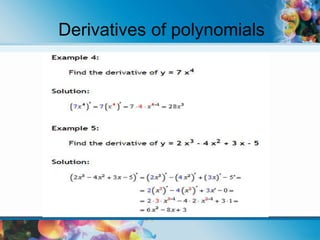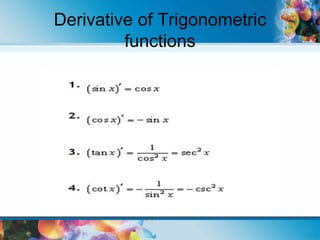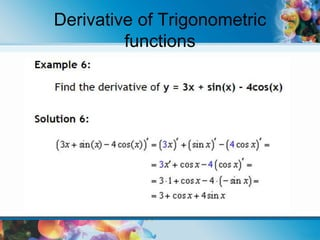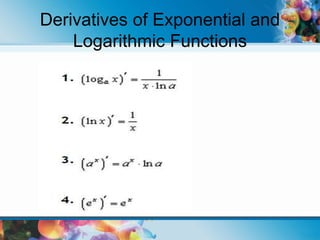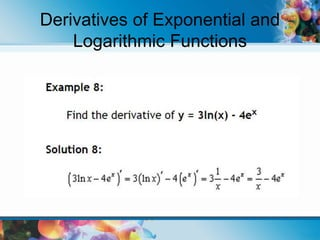1 sur 43

### mathspresentation-160419194459.pdf

• 2. What is derivative? • The derivative of a function of a real variable measures the sensitivity to change of a quantity (a function or dependent variable) which is determined by another quantity (the independent variable). • For example, the derivative of the position of a moving object with respect to time is the object's velocity: this measures how quickly the position of the object changes when time is advanced.
• 3. What is differentiation? • The process of finding a derivative is called differentiation. • The reverse process is called antidifferentiation. • The fundamental theorem of calculus states that antidifferentiation is the same as integration. • Differentiation is also known as the process to find rate of change • Derivative tells us slope of function at any point.
• 4. As it is also rate of change • The rate of change of a function is expressed as a ratio between a change in one variable relative to a corresponding change in another. • Rate of change is also given by limit value.
• 5. Average rate of change • The Average Rate of Change is defined as the ratio of the difference in the function f(x) as it changes from 'a' to 'b' to the difference between 'a' and 'b'. The average rate of change is denoted as A(x). • And is given as the formula • A(x) = 𝑓 𝑏 −𝑓(𝑎) 𝑏−𝑎 • The Average Rate of Change Formula calculates the slope of a line or a curve on a given range.
• 6. Average rate of change-Ex Calculate the average rate of change of a function, f(x) = 3x + 5 as x changes from a to b ? Solution: Given, f(x) = 3x + 5 a = 3 , b = 6 Putting the values f(3) = 3(3) + 5 :f(6) = 3(6) + 5 f(3)=14 : f(6)=23 The average rate of change is, A(x) = 𝒇 𝒃 −𝒇 𝒂 𝒃−𝒂 A(x) = 𝟐𝟑−𝟏𝟒 𝟑 = 𝟗 𝟑 A(x) = 3
• 7. Notations for derivatives Leibniz's notation. 𝒅𝒚 𝒅𝒙 , 𝒅𝒇 𝒅𝒙 x Lagrange's notation. f’ f” Euler's notation. 𝑫𝒙𝒀 𝑶𝒓 𝑫𝒙𝑭(𝒙)
• 8. Derivative Notation A Function f(x) y X2 + 1 Newton Form f ’(x) y ’ (X2 + 1)’ Leibniz Form (f(x)) (y) (X2 + 1) d dx d dx d dx or or df dx df dx Derivative Notation
• 9. Notation There are lots of ways to denote the derivative of a function y = f(x). f’(x) the derivative of f the derivative of f with y’ y prime respect to x. the derivative of y the derivative of f at x with respect to x. dx dy dx df ) (x f dx d
• 16. Examples Product rule: • This is used when differentiating a product of two functions. Differentiate x(x² + 1) • let u = x and v = x² + 1 • 𝑑𝑢 𝑑𝑥 = 1 𝑑𝑣 𝑑𝑥 = 2𝑥
• 19. Examples Power Rule: "what is the derivative of 𝒙𝟔 ?" • We can use the Power Rule, where n=6: • 𝑥𝑛 = 𝑛𝑥𝑛−1 • So, • 𝑥6 = 6𝑥6−1 = 6𝑥5
• 20. Chain rule A special rule, the chain rule, exists for differentiating a function of another function. In order to differentiate a function of a function, y = f(g(x)), That is to find 𝑑𝑦 𝑑𝑥 , we need to do two things: 1. Substitute u = g(x). This gives us y = f(u) Next we need to use a formula that is known as the Chain Rule. 2. Chain Rule 𝒅𝒚 𝒅𝒙 = 𝒅𝒚 𝒅𝒖 × 𝒅𝒖 𝒅𝒙
• 21. Chain Rule Example Chain rule: If 𝑦 = 3 2𝑥 − 1 2𝑢 = 2𝑥 − 1 • Find 𝑑𝑦 𝑑𝑥 Solution: 𝑦 = 3 2𝑥 − 1 2 , 𝑦 = 3 𝑢 2 • Taking derivative to both side 𝒅𝒚 𝒅𝒖 = 6𝑢 -------(i 𝑢 = 2𝑥 − 1 • Taking derivative to both side 𝒅𝒖 𝒅𝒙 = 2
• 22. Chain rule: • Using chain rule • 𝑑𝑦 𝑑𝑥 = 𝑑𝑦 𝑑𝑢 . 𝑑𝑢 𝑑𝑥 • 𝑑𝑦 𝑑𝑥 = 6𝑢. 2 • = 12u ∴ 𝑢 = 2𝑥 − 1 =12(2x-1) =24x-12 Chain Rule Example
• 24. Higher derivatives. 1st ,2nd 3rd • Any derivative beyond the first derivative can be referred to as a higher order derivative. • The derivative of the function f(x) may be denoted by f’(x) • Its double (or "second") derivative is denoted by f ’’(x). • This is read as "f double prime of x," or "The second derivative of f(x)."
• 25. Higher derivatives. Notations: Let f(x) be a function in terms of x. The following are notations for higher order derivatives.
• 28. Partial derivatives Partial derivatives are defined as derivatives of a function of multiple variables when all but the variable of interest are held fixed during the differentiation
• 29. Partial derivatives:  The partial derivative of f is with respect to its variable.  Here ∂ is a rounded d called the partial derivative symbol. To distinguish it from the letter d, ∂ is sometimes pronounced "der", "del", or "partial" instead of "dee"
• 31. Applications of partial derivatives: • Derivatives are constantly used in everyday life to help measure how much something is changing. They're used by the government in population censuses, various types of sciences, and even in economics..
• 32. Applications of partial derivatives: • Derivatives in physics. You can use derivatives a lot in Newton law of motion where the velocity is defined as the derivative of the position over time and the acceleration, the derivative of the velocity over time. • Derivatives in chemistry. One use of derivatives in chemistry is when you want to find the concentration of an element in a product.
• 33. Concave Up • The derivative of a function gives the slope • When the slope continually increases, the function is concave upward. • Taking the second derivative actually tells us if the slope continually increases or decreases. • When the second derivative is positive, the function is concave upward. • f ''(x) > 0 for x > 0;
• 35. Concave down: • When the slope continually decreases, the function is concave downward. • When the second derivative is negative, the function is concave downward. • f ''(x) < 0 for x < 0.
• 41. Derivatives of Exponential and Logarithmic Functions
• 42. Derivatives of Exponential and Logarithmic Functions
• 43. • Thank You So much for Listening • Any Question?